Arduino

# Active Low Pass Filter RC applied in Projects with ArduinoMay 07, 2020 by Lima Moreira
Share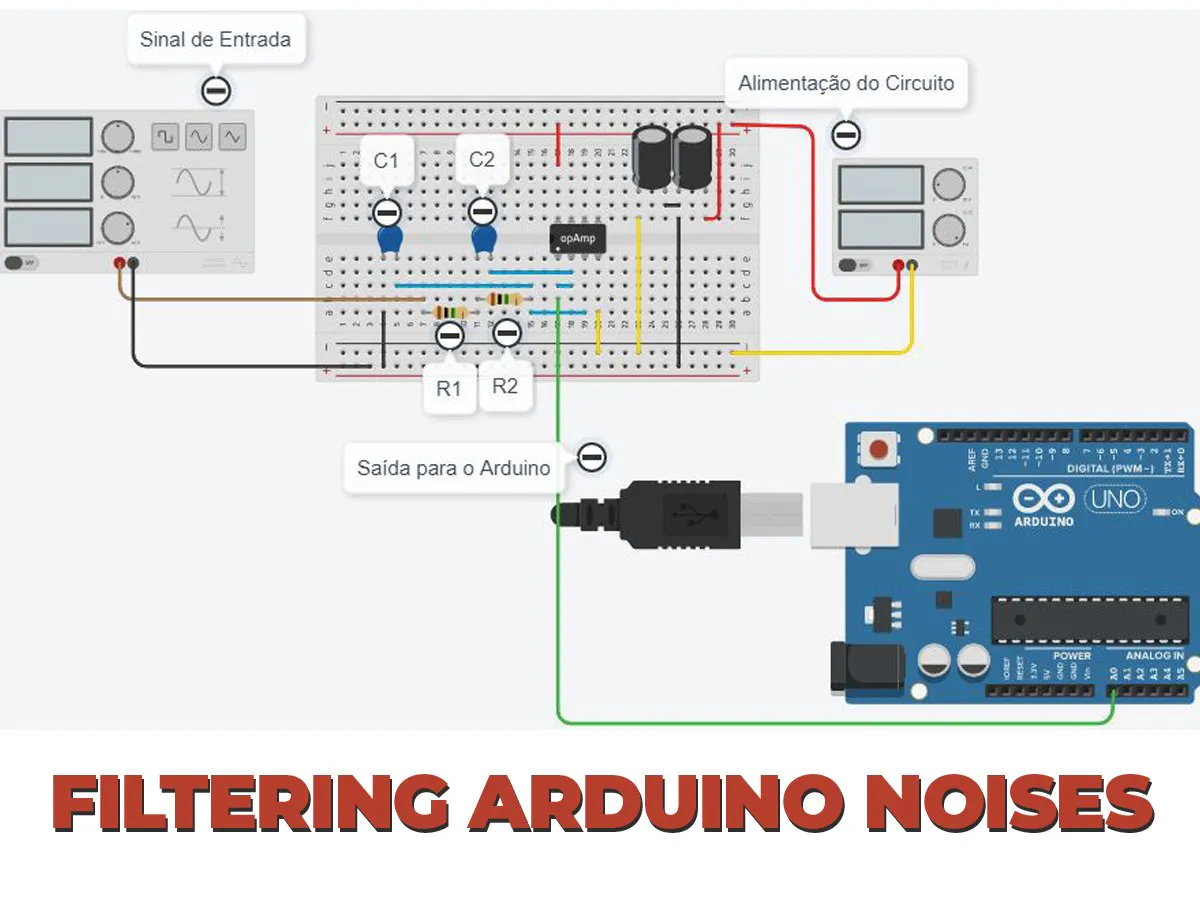You'll learn how to build your Active Low Pass Filter RC applied in Projects with Arduino

### The project of the low pass filter RC

The low pass filter is excellent electronic circuits to filter out parasitic signals from your projects. A common problem in projects with Arduino and systems with sensors working close to power circuits is the presence of “parasitic” signals.

They can be caused by vibration or magnetic fields in the same area as the sensor.

These signals, which are mostly of high frequency, cause disturbance at the time of reading and, consequently, erroneous readings occur in the automation system. A common example is the starting of a machine that requires a high initial current.

This will cause the generation of high-frequency noise in several elements that are connected to the electrical network, including sensors.

To prevent these noises from affecting the system, filters are used between the sensor element and the system that reads it.

What are passive and active filters?

### What are passive and active filters?

Filters are circuits that can “clean” a signal, separate unwanted signals, to avoid reading values that do not match reality.

Filters can be of two types: passive and active.

Passive Filters

Filters can be passive, which are the simplest, as they consist only of resistors and capacitors.

Active Filters

Active filters, in addition to resistors and capacitors, use amp-ops to improve filtering, and digital filters, which are used in processors and microcontrollers.

• Understand how low pass filter work;
• Configure the hardware of the low pass filter with a cutoff frequency of 100 Hz using an operational amplifier LM358;
• Calculate the values of the passive components of the circuit;
• Assemble a low pass filter NextPCB.

Below, we present the process of developing the active low pass filter for our circuits with Arduino.

### Development of the active low pass filter RC circuit

In this project an active low pass filter will be developed with NEXTPCB - Printed Circuit Board, that is, it allows us to pass low frequencies. The frequency range to be chosen depends on the operation of the circuit.

For this article we will use an active low pass filter, as they are used for frequencies below 1MHz, and, in addition, signal amplification can be done, as an operational amplifier will be used in this circuit.

Therefore, based on this project, the central focus will be on the development of the active low pass filter circuit and its symmetrical supply circuit. Figure 1 illustrates the hardware of this circuit.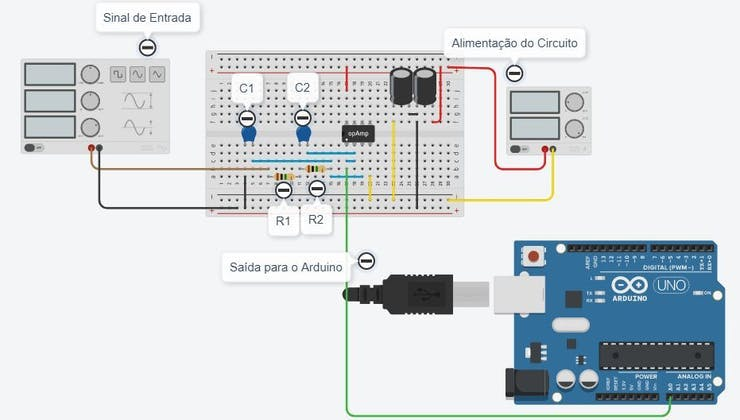Figure 1 - Low Pass Filter RC circuit constructed in TinkerCAD.

The low pass filter RC circuit constructed in TinkerCAD can be accessed at the following link: https://www.tinkercad.com/things/0H8Wq5NJLm0-filtro-ativo

As mentioned, we used Arduino in this project in order to acquire the signal from a sensor. Thus, the low pass filter RC circuit in the figure above we have 3 important parts:

• The signal generator,
• The active filter and;
• Arduino for collecting sensor data.

The signal generator is responsible for simulating the functioning of a sensor and transmitting the signal to the Arduino. This signal is then filtered through the low pass filter RC and, subsequently, the filtered signal is read and processed by Arduino.

Thus, to perform the assembly of the low pass filter RC we will need the following electronic components:

• 2 Resistors;
• 2 ceramic capacitors;
• 2 Electrolytic capacitors;
• Operational Amplifier LM358;
• Power terminals or 9V battery;

Next, we present the calculation of the values of the resistors and capacitors of the circuit. The calculation of these components is based on the low pass filter cutoff frequency of the active filter.

### Resistor and Capacitor Calculations

For the proposed circuit, we will use a low pass filter cutoff frequency of 100Hz. In this way, the circuit will allow frequencies to pass below 100Hz and above 100Hz, the signal will decrease exponentially.

Therefore, for the calculation of capacitors, we have: Initially, it is enough to define a value of C1, in which case a commercial value of 1 to 100nF can be defined.

Next, we performed the calculation of capacitor C2 according to the equation below.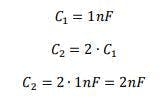Equation 1 - Calculation of the capacitor value.

Then use the formula below to calculate the value of R1 and R2. The formula can be used to project the value of the two resistors. Next, see the calculation performed.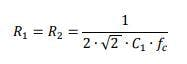Equation 2 - Calculation of the resistor value.

Where f*C is the low pass filter cutoff frequency, that is, above that frequency, the gain of this signal will decrease. The f*C value for this system will be 100 Hz.

Therefore, we have the following resistor value for R1 and R2.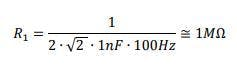Equation 3 - R1 Resistor Value.

From the values obtained for the resistors and capacitor of the project, we must then develop the power supply circuit for the active filter. For this type of filter, we need to use a symmetrical power supply and, next, we will present the supply circuit.

### The Power Supply

The required power for this circuit is a symmetrical power supply. If you do not have a symmetric power supply, assemble a circuit using capacitors powered by a simple power supply.

However, the voltage value of the power supply must be greater than 10V, as the value of the symmetric source will be divided by 2.

Figure 2 shows the circuit of the power supply.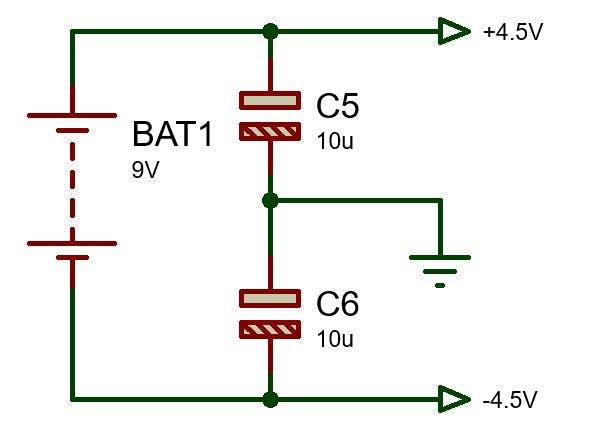Figure 2 - Auxiliary circuit to transform simple voltage into symmetrical voltage.

This circuit is already in the electronic diagram in Figure 1, since a common non-symmetric source is used. After designing the active filter circuit and its supply circuit, we developed an electronic filter module to be used in your projects with Arduino or in other projects that need a filter for this purpose.

Next, we will present the structure of the electronic scheme and the design of the developed electronic board.

### The printed circuit board of the Active Low Pass Filter RC

In order to make the electronic printed circuit board - NEXTPCB, the electronic schematic of the circuit was developed. The electronic schematic of the Active Low Pass Filter RC is shown in Figure 3.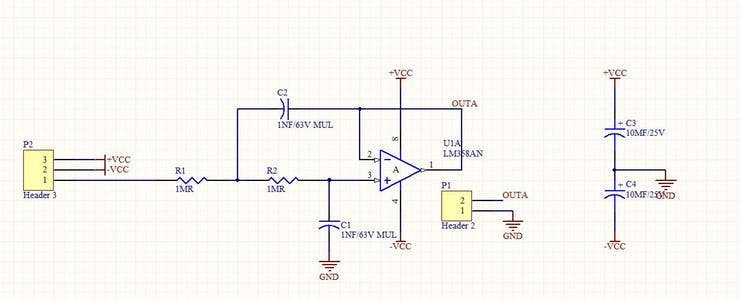Figure 3 - Electronic schematic project of the Low Pass filter RC.

Then, the scheme was exported to the PCB Design of the Altium software and the following board was designed, as shown in Figure 4.

Three pins were used to supply the circuit and input signal and two pins at the output. The two pins are used for the output of the filtered signal and the GND of the circuit.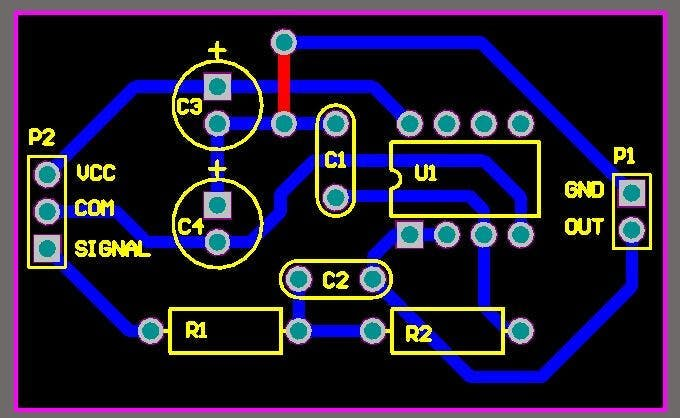Figure 4 - Layout of the Printed Circuit Board.

After designing the layout of the PCB, the 3D design of the printed circuit board was generated and presented in Figure 5.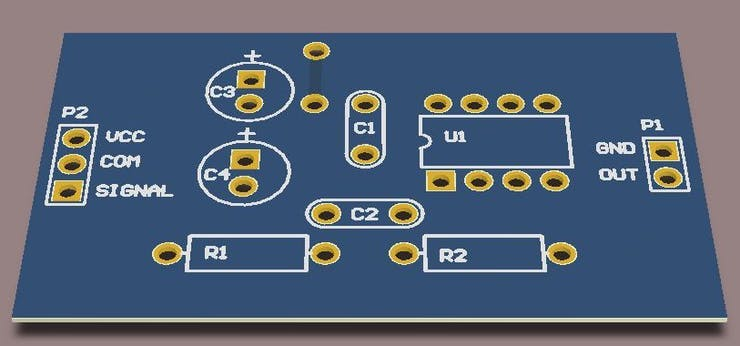Figure 5 - 3D Printed Circuit Board.

From the PCB project, you can use this module and apply it to your project with Arduino. This way, certain parasitic signals will be canceled and your project will work without risk of errors in the signal reading.

### Conclusion

This active low pass filter RC circuit can be widely used for filtering the power of the Arduino, filtering the signals of serial communication, as in radio frequency, which usually has many signals that usually cause interference in the serial communication, provided that the value of the cutoff frequency is changed.

A tip after assembling this circuit is to make the connection closer to the Arduino, as a good part of the interference is in the distance between the sensor and the microcontroller, and in most cases, the microcontroller cannot be very close, because the location of the sensor can be harmful to the Arduino.

In addition, to have a more continuous signal, just change the low pass filter cutoff frequency to a lower frequency, this will change the values of the resistors and capacitors. It also has its advantages of creating a gain in the signal, if the signal is low.

### Important Information

All files can be accessed in the following link: Files of the Printed Circuit Board